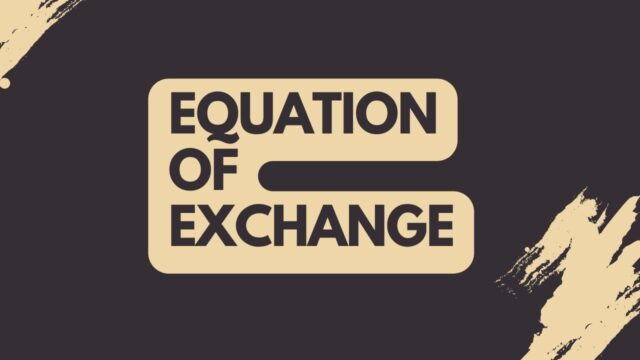# Equation of Exchange## What is the equation of exchange and what does it mean for economics

The equation of exchange is a mathematical formula that is used to quantify the relationship between money supply and money demand in an economy. The formula is M x V = P x Q, where M is the money supply, V is the velocity of money, P is the price level, and Q is the quantity of goods and services produced. The velocity of money is a measure of how often a unit of currency is traded in a given period of time. The quantity of goods and services produced is also known as economic output.

The equation of exchange can be used to determine whether an increase in the money supply will lead to inflation or not. If the money supply increases but the velocity of money remains constant, then prices will rise and there will be inflation. However, if the velocity of money increases at the same time as the money supply, then there will be no change in prices and no inflation. The equation of exchange is thus a useful tool for economists to understand how changes in the money supply can impact inflation.

## How has the equation of exchange been used throughout history

The equation of exchange has been used by economists throughout history to understand how changes in the money supply can impact economic activity. For example, in the early 20th century, economist Irving Fisher used the equation to argue that increases in the money supply would lead to higher inflation. More recently, economists have used the equation to better understand how changes in the money supply can impact economic activity in different ways. For instance, some economists have argued that increases in the money supply can lead to both higher inflation and higher economic growth. Others have argued that increases in the money supply can lead to higher inflation but lower economic growth. Ultimately, the equation of exchange provides a helpful way for economists to think about how changes in the money supply can impact economic activity.

## How can the equation of exchange be used to understand economic trends

The equation of exchange is a simple yet powerful tool that can help to understand a variety of economic trends. In its most basic form, the equation states that the money supply multiplied by the velocity of money is equal to the price level multiplied by the quantity of transactions. This equation can be used to understand inflation, for example. If the money supply increases but the velocity of money remains unchanged, then prices will rise as more money is chasing the same number of goods and services.

The equation can also be used to understand economic growth. If the quantity of transactions increases but the money supply remains unchanged, then prices will rise as demand outstrips supply. In other words, economic growth can lead to inflationary pressures. By understanding the equation of exchange, we can gain valuable insights into a wide range of economic trends.

## What are some criticisms of the equation of exchange

The model has come under criticism in recent years for its simplification of a complex economic phenomenon. One major criticism is that the equation assumes that all money is spent, which is not the case in reality. Money can be saved or invested, which means that it is not always available to be exchanged for goods and services. Additionally, the model does not take into account the different velocity of money in different sectors of the economy. For example, money may circulate more quickly in the stock market than in the housing market. As a result, the equation of exchange may not accurately reflect the true relationship between money supply and inflation.

## How might the equation of exchange be improved

The equation of exchange can be improved by adding variables that measure important economic activity, such as productivity, population growth, and inflation. Additionally, the equation of exchange can be modified to reflect different economic theories, such as Keynesian economics and monetarist economics. By improving the equation of exchange, economists can gain a better understanding of how the money supply affects economic activity. Additionally, policymakers can use the improved equation of exchange to make more informed decisions about monetary policy.

## What does the future hold for the equation of exchange

The future of the equation of exchange is shrouded in uncertainty. The equation has been a reliable predictor of inflation for centuries, but its ability to continue doing so is now being called into question. Many factors, such as global economic conditions and the actions of central banks, can affect the rate of inflation. As a result, the equation of exchange may need to be modified in order to take these factors into account. Additionally, the increasing use of electronic currency could lead to further changes in the way that inflation is calculated. Despite these challenges, the equation of exchange remains a valuable tool for understanding changes in prices. With some modifications, it is likely to remain an important part of economics for years to come.